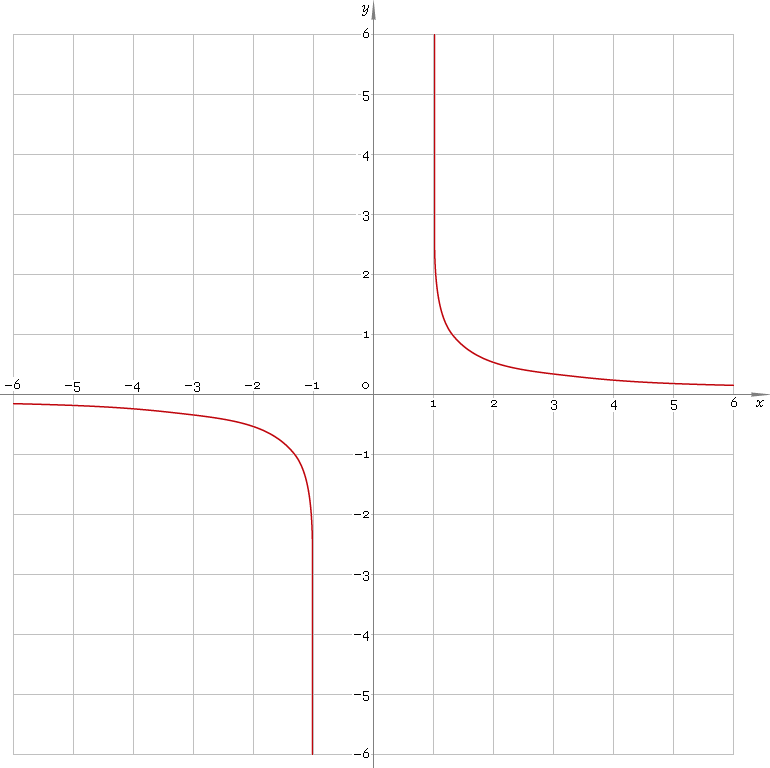The Art of Mathematics

# arcoth or arcth — arc-hyperbolic cotangent function

## 1. Definition

Arc-hyperbolic cotangent is inverse of hyperbolic cotangent function.

arcothxcothinvx

With the help of natural logarithm it can be represented as:

arcothx ≡ ln[(1 + x) /(x − 1)] /2

## 2. Graph

Arc-hyperbolic cotangent is antisymmetric function defined everywhere on real axis, except the range [−1, 1] — so its domain is (−∞, −1)∪(1, +∞). Points x = ±1 are singular ones. Function graph is depicted below — fig. 1.Fig. 1. Graph of the arc-hyperbolic cotangent function y = arcothx.

Function codomain is all real axis, except 0: (−∞, 0)∪(0, +∞).

## 3. Identities

Property of antisymmetry:

arcoth−x = −arcothx

Reciprocal argument:

arcoth(1/x) = artanhx

Sum and difference:

arcothx + arcothy = arcoth[(1 + xy) /(x + y)]
arcothx − arcothy = arcoth[(1 − xy) /(xy)]

## 4. Derivative and indefinite integral

Arc-hyperbolic cotangent derivative:

arcoth′x = 1 /(1 − x2)

Indefinite integral of the arc-hyperbolic cotangent:

∫ arcothx dx = x arcothx + ln|x2 − 1| /2 + C

where C is an arbitrary constant.

## 5. How to use

To calculate arc-hyperbolic cotangent of the number:

``arcoth(−2);``

To get arc-hyperbolic cotangent of the complex number:

``arcoth(−2+i);``

To get arc-hyperbolic cotangent of the current result:

``arcoth(rslt);``

To get arc-hyperbolic cotangent of the number z in calculator memory:

``arcoth(mem[z]);``

## 6. Support

Arc-hyperbolic cotangent of the real argument is supported in free version of the Librow calculator.

Arc-hyperbolic cotangent of the complex argument is supported in professional version of the Librow calculator.